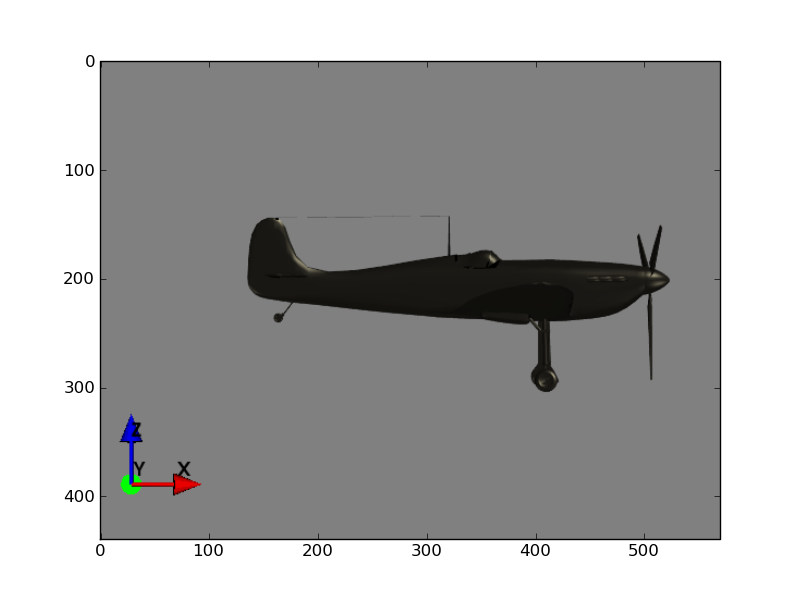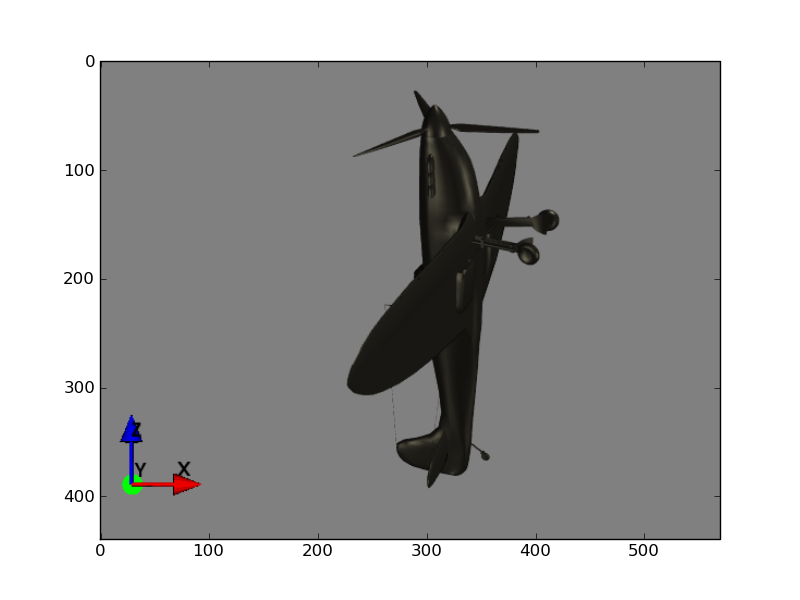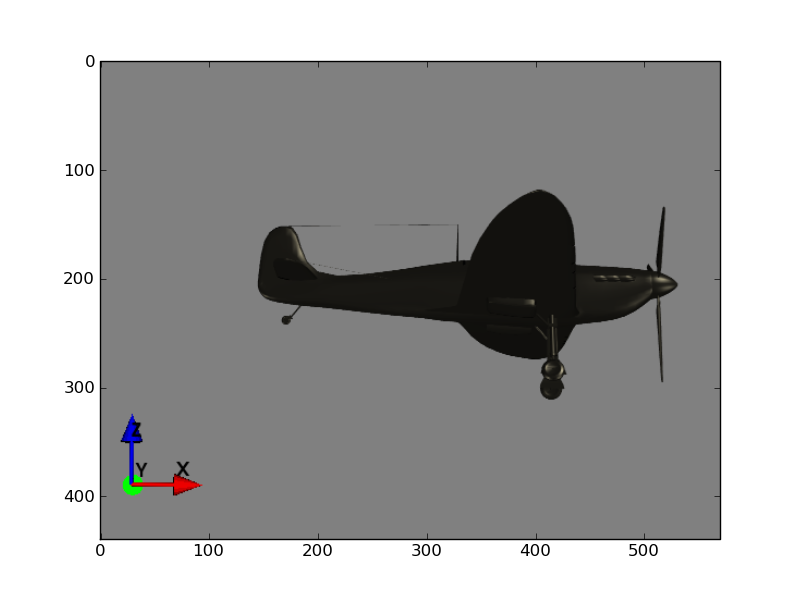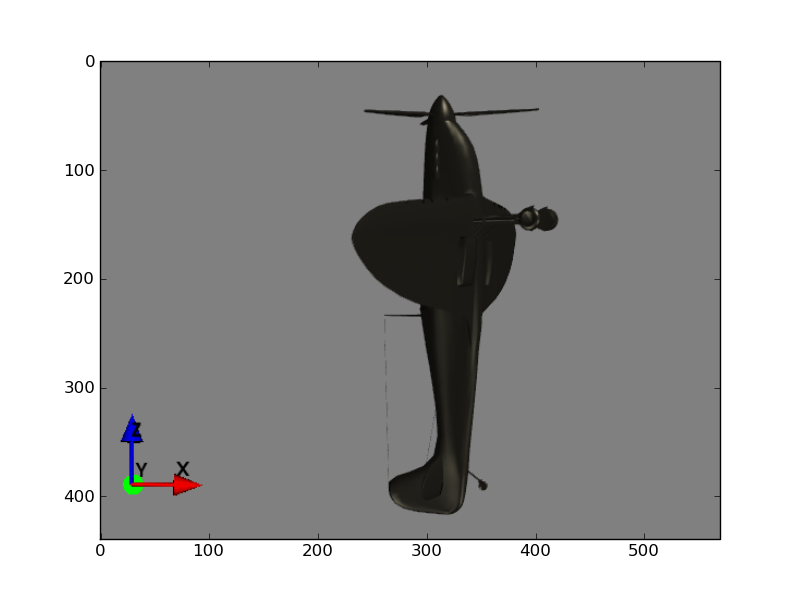# Gimbal lock¶

Euler angles have a major deficiency, and that is, that it is possible, in some rotation sequences, to reach a situation where two of the three Euler angles cause rotation around the same axis of the object. In the case below, rotation around the $$x$$ axis becomes indistinguishable in its effect from rotation around the $$z$$ axis, so the $$z$$ and $$x$$ axis angles collapse into one transformation, and the rotation reduces from three degrees of freedom to two.

## Example¶

Imagine that we are using the Euler angle convention of starting with a rotation around the $$x$$ axis, followed by the $$y$$ axis, followed by the $$z$$ axis.

Here we see a Spitfire aircraft, flying across the screen. The $$x$$ axis is left to right (tail to nose), the $$y$$ axis is from the left wing tip to the right wing tip (going away from the screen), and the $$z$$ axis is from bottom to top:We want to rotate the aircraft to look something like this:We might start by doing an x rotation, making a slight roll with the left wing tilting down (rotation about $$x$$) like this:Let’s say that the x rotation is -0.2 radians.

Then we do a pitch so we are pointing straight up (rotation around $$y$$ axis). This is a rotation by $$-\pi/2$$ radians.To get to our desired position from here, we need to do a turn of something like 0.2 radians of the nose towards the viewer (and the tail away from the viewer). All we have left is our z rotation (rotation around the $$z$$ axis. Unfortunately, the current result of a rotation around the $$z$$ axis has now become the same as a previous rotation around the $$x$$ axis. To see this, look at the result of the rotation around the $$y$$ axis. Notice that the $$x$$ axis, as was, is now aligned with the $$z$$ axis, as it is now. Rotating around the $$z$$ axis will have exactly the same effect as adding an extra rotation around the $$x$$ axis at the beginning. That means that, when there is a $$y$$ axis rotation that rotates the $$x$$ axis onto the $$z$$ axis (a rotation of $$\pm\pi/2$$ around the $$y$$ axis) - the $$x$$ and $$y$$ axes are “locked” together.

This does not mean that we cannot do the rotations we need, only that we can’t do them by starting with the most obvious x and y rotations. In fact what we will have to do is first rotate around x by $$\pi/2 - 0.2$$ radians, then do a y rotation of $$-\pi/2 + 0.2$$ radians, and finally a z rotation of $$-\pi/2$$ radians. See the code below for the details.

## Mathematics of gimbal lock¶

We see gimbal lock for this type of Euler axis convention, when $$\cos(\beta) = 0$$, where $$\beta$$ is the angle of rotation around the $$y$$ axis. By “this type of convention” we mean using rotation around all 3 of the $$x$$, $$y$$ and $$z$$ axes, rather than using the same axis twice - e.g. the physics convention of $$z$$ followed by $$x$$ followed by $$z$$ axis rotation (the physics convention has different properties to its gimbal lock).

We can show how gimbal lock works by creating a rotation matrix for the three component rotations. Recall that, for a rotation of $$\alpha$$ radians around $$x$$, followed by a rotation $$\beta$$ around $$y$$, followed by rotation $$\gamma$$ around $$z$$, the rotation matrix $$R$$ is:

$\begin{split}R = \left[\begin{matrix}\cos{\left (\beta \right )} \cos{\left (\gamma \right )} & \sin{\left (\alpha \right )} \sin{\left (\beta \right )} \cos{\left (\gamma \right )} - \sin{\left (\gamma \right )} \cos{\left (\alpha \right )} & \sin{\left (\alpha \right )} \sin{\left (\gamma \right )} + \sin{\left (\beta \right )} \cos{\left (\alpha \right )} \cos{\left (\gamma \right )}\\\sin{\left (\gamma \right )} \cos{\left (\beta \right )} & \sin{\left (\alpha \right )} \sin{\left (\beta \right )} \sin{\left (\gamma \right )} + \cos{\left (\alpha \right )} \cos{\left (\gamma \right )} & - \sin{\left (\alpha \right )} \cos{\left (\gamma \right )} + \sin{\left (\beta \right )} \sin{\left (\gamma \right )} \cos{\left (\alpha \right )}\\- \sin{\left (\beta \right )} & \sin{\left (\alpha \right )} \cos{\left (\beta \right )} & \cos{\left (\alpha \right )} \cos{\left (\beta \right )}\end{matrix}\right]\end{split}$

In our case the y rotation $$\beta = -\pi / 2, \cos(\beta) = 0, \sin(\beta) = -1$$:

$\begin{split}R = \left[\begin{matrix}0 & - \sin{\left (\alpha \right )} \cos{\left (\gamma \right )} - \sin{\left (\gamma \right )} \cos{\left (\alpha \right )} & \sin{\left (\alpha \right )} \sin{\left (\gamma \right )} - \cos{\left (\alpha \right )} \cos{\left (\gamma \right )}\\0 & - \sin{\left (\alpha \right )} \sin{\left (\gamma \right )} + \cos{\left (\alpha \right )} \cos{\left (\gamma \right )} & - \sin{\left (\alpha \right )} \cos{\left (\gamma \right )} - \sin{\left (\gamma \right )} \cos{\left (\alpha \right )}\\1 & 0 & 0\end{matrix}\right]\end{split}$

From the angle sum and difference identities (see also geometric proof, Mathworld treatment) we remind ourselves that, for any two angles $$\alpha$$ and $$\beta$$:

\begin{align}\begin{aligned}\sin(\alpha \pm \beta) = \sin \alpha \cos \beta \pm \cos \alpha \sin \beta \,\\\cos(\alpha \pm \beta) = \cos \alpha \cos \beta \mp \sin \alpha \sin \beta\end{aligned}\end{align}

We can rewrite $$R$$ as:

$\begin{split}R = \left[\begin{matrix}0 & - W_{1} & - W_{2}\\0 & W_{2} & - W_{1}\\1 & 0 & 0\end{matrix}\right]\end{split}$

where:

\begin{align}\begin{aligned}W_1 = \sin{\left (\alpha \right )} \cos{\left (\gamma \right )} + \sin{\left (\gamma \right )} \cos{\left (\alpha \right )} = \sin(\alpha + \gamma) \,\\W_2 = - \sin{\left (\alpha \right )} \sin{\left (\gamma \right )} + \cos{\left (\alpha \right )} \cos{\left (\gamma \right )} = \cos(\alpha + \gamma)\end{aligned}\end{align}

We immediately see that $$\alpha$$ and $$\gamma$$ are going to lead to the same transformations - the mathematical expression of the observation on the spitfire above, that rotation around the $$x$$ axis is equivalent to rotation about the $$z$$ axis.

It’s easy to do the same set of reductions for the case where $$\sin(\beta) = 1$$; see http://www.gregslabaugh.name/publications/euler.pdf.

## The example in code¶

Here is what our gimbal lock looks like in code:

>>> import numpy as np
>>> np.set_printoptions(precision=3, suppress=True)  # neat printing
>>> from transforms3d.euler import euler2mat, mat2euler
>>> x_angle = -0.2
>>> y_angle = -np.pi / 2
>>> z_angle = -0.2
>>> R = euler2mat(x_angle, y_angle, z_angle, 'sxyz')
>>> R
array([[ 0.   ,  0.389, -0.921],
[-0.   ,  0.921,  0.389],
[ 1.   , -0.   ,  0.   ]])


This isn’t the transformation we actually want because of the gimbal lock. The gimbal lock means that x_angle and z_angle result in rotations about the same axis of the object. So, we can add something to the x_angle and subtract the same value from z_angle to get the same result:

>>> R = euler2mat(x_angle + 0.1, y_angle, z_angle - 0.1, 'sxyz')
>>> R
array([[ 0.   ,  0.389, -0.921],
[-0.   ,  0.921,  0.389],
[ 1.   , -0.   ,  0.   ]])


In fact, we could omit the z rotation entirely and put all the rotation into the original x axis rotation and still get the same rotation matrix:

>>> R_dash = euler2mat(x_angle + z_angle, y_angle, 0, 'sxyz')
>>> np.allclose(R, R_dash)
True


So, there is no future in doing our transformations starting with this x and y rotation, if we are rotating with this axis order. We can get the transformation we actually want by doing the rotations in the order x, then z then y, like this:

>>> R = euler2mat(x_angle, z_angle, y_angle, 'sxzy')
>>> R
array([[ 0.   ,  0.199, -0.98 ],
[-0.199,  0.961,  0.195],
[ 0.98 ,  0.195,  0.039]])


We can get this same transformation using our original x, y, z rotation order, but using different rotation angles:

>>> x_dash, y_dash, z_dash = mat2euler(R, 'sxyz')
>>> np.array((x_dash, y_dash, z_dash))  # np.array for print neatness
array([ 1.371, -1.371, -1.571])
>>> R = euler2mat(x_dash, y_dash, z_dash, 'sxyz')
>>> R
array([[ 0.   ,  0.199, -0.98 ],
[-0.199,  0.961,  0.195],
[ 0.98 ,  0.195,  0.039]])